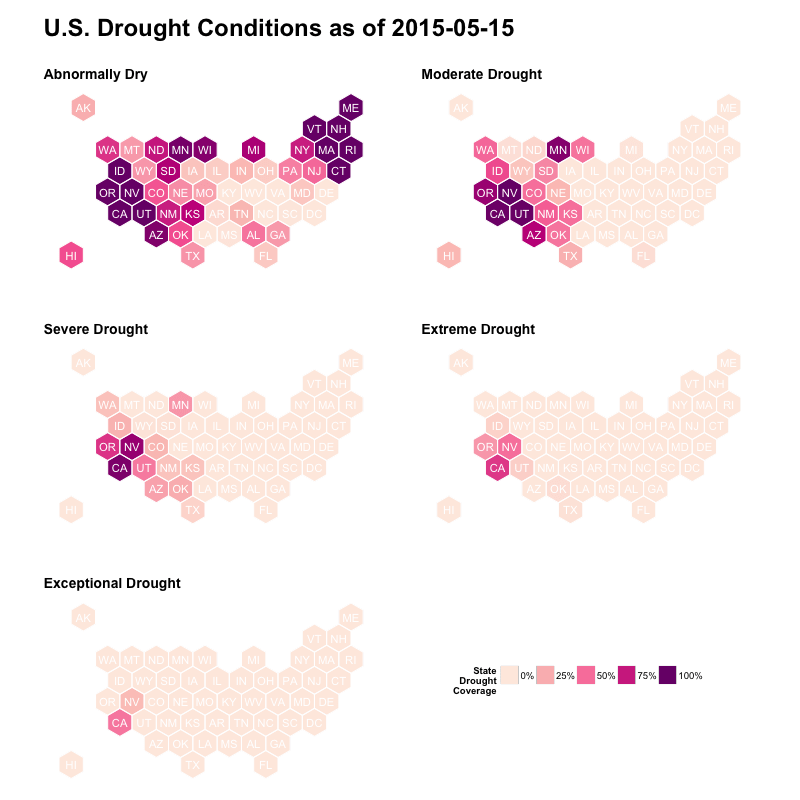# U.S. Drought Monitoring With Hexbin State Maps in R

On the news, today, of the early stages of drought hitting the U.S. northeast states I decided to springboard off of yesterday’s post and show a more practical use of hexbin state maps than the built-in (and still purpose unknown to me) “bees” data.

The U.S. Drought Monitor site supplies more than just a pretty county-level map. There’s plenty of data and you can dynamically retrieve just data tables for the whole U.S., U.S. states and U.S. counties. Since we’re working with state hexbins, we just need the state-level data. Drought levels for all five stages are reported per-state, so we can take all this data and created a faceted/small-multiples map based on it.

This builds quite a bit on the previous work, so you’ll see some familiar code. Most of the new code is actually making the map look nice (the great part about this is that once you have the idiom down, it’s just a matter of running the script each day vs a billion mouse clicks). The other bit of new code is the data-retrieval component:

``````library(readr)
library(tidyr)
library(dplyr)

intensity <- c(D0="Abnormally Dry", D1="Moderate Drought", D2="Severe Drought",
D3="Extreme Drought", D4="Exceptional Drought")

today <- format(Sys.Date(), "%Y%m%d")

gather(drought_level, value, D0, D1, D2, D3, D4) %>%
mutate(intensity=factor(intensity[drought_level],
levels=as.character(intensity), ordered=TRUE)) -> drought``````

This:

• sets up a fast way to add the prettier description of the drought levels (besides D0, D1, etc)
• dynamically uses today’s date as the parameter for the URL we read with `read_csv` (from the `readr` package)
• covert the data from wide to long

The `ggplot` code will facet on the intensity level to make the overall map:

``````library(rgdal)
library(rgeos)
library(ggplot2)
library(tidyr)
library(dplyr)
library(grid)

centers <- cbind.data.frame(data.frame(gCentroid(us, byid=TRUE), id=us@data\$iso3166_2))

us_map <- fortify(us, region="iso3166_2")

intensity <- c(D0="Abnormally Dry", D1="Moderate Drought", D2="Severe Drought",
D3="Extreme Drought", D4="Exceptional Drought")

today <- format(Sys.Date(), "%Y%m%d")

gather(drought_level, value, D0, D1, D2, D3, D4) %>%
mutate(intensity=factor(intensity[drought_level],
levels=as.character(intensity), ordered=TRUE)) -> drought

gg <- ggplot()
gg <- gg + geom_map(data=us_map, map=us_map,
aes(x=long, y=lat, map_id=id),
color="white", size=0.5)
gg <- gg + geom_map(data=drought, map=us_map,
aes(fill=value, map_id=State))
gg <- gg + geom_map(data=drought, map=us_map,
aes(map_id=State),
fill="#ffffff", alpha=0, color="white",
show_guide=FALSE)
gg <- gg + geom_text(data=centers, aes(label=id, x=x, y=y), color="white", size=4)
gg <- gg + scale_fill_distiller(name="State\nDrought\nCoverage", palette="RdPu", na.value="#7f7f7f",
labels=sprintf("%d%%", c(0, 25, 50, 75, 100)))
gg <- gg + coord_map()
gg <- gg + facet_wrap(~intensity, ncol=2)
gg <- gg + labs(x=NULL, y=NULL, title=sprintf("U.S. Drought Conditions as of %s\n", Sys.Date()))
gg <- gg + theme_bw()
gg <- gg + theme(plot.title=element_text(face="bold", hjust=0, size=24))
gg <- gg + theme(panel.border=element_blank())
gg <- gg + theme(panel.margin=unit(3, "lines"))
gg <- gg + theme(panel.grid=element_blank())
gg <- gg + theme(axis.ticks=element_blank())
gg <- gg + theme(axis.text=element_blank())
gg <- gg + theme(strip.background=element_blank())
gg <- gg + theme(strip.text=element_text(face="bold", hjust=0, size=14))
gg <- gg + theme(legend.position=c(0.75, 0.15))
gg <- gg + theme(legend.direction="horizontal")
gg <- gg + theme(legend.title.align=1)

png(sprintf("%s.png", today), width=800, height=800)
print(gg)
dev.off()``````Now, you can easily animate these over time to show the progression/regression of the drought conditions. If you're sure your audience can work with SVG files, you can use those for very crisp/sharp maps (and even feed it to D3 or path editing tools). If you have an example of how you're using hexbin choropleths, drop a note in the comments. The code from above is also on github.Amazon Author Page

This site uses Akismet to reduce spam. Learn how your comment data is processed.